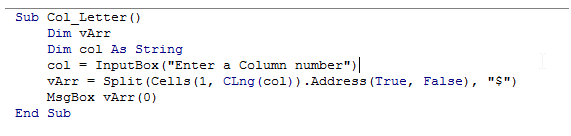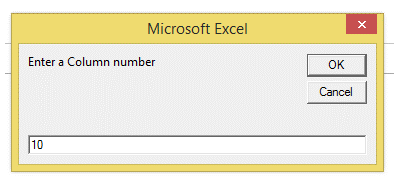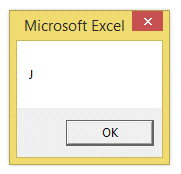## Vba cell address to column number in Excel

This topic, as the name implies, deals with finding column letters from the column number.

This sort of requirement arises, when a data is given and its location has to be found and used in the program.

for example, let's say the data is a string, which is present in Cell F8.

We can find the column number using a For loop.Now that, we have the column number.

Now, to use this address in the program, it has to be converted to a named address location.

In multi-module programming, this requirement often arises.

The following example demonstrates this:

1. Sub Col_Letter()
2. Dim vArr
3. Dim col As String
4. col = InputBox("Enter a Column number")
5. vArr = Split(Cells(1, CLng(col)).Address(True, False), "\$")
6. MsgBox vArr(0)
7. End Sub

The above example obtains a column number from the user and returns the corresponding Column letter.

The Screenshot of the VBA Editor is as shown below:The Output is as shown: